# Difference-element-in-K-theory

An element of the group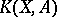(where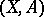is a pair of spaces andis usually supposed to be a finite cellular space, whileis a cellular subspace of it), constructed from a triple, whereandare vector bundles of the same dimension overand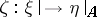is an isomorphism of vector bundles (hereis the part of the vector bundleoverlocated above the subspace). The construction of a difference element can be carried out in the following way. First one supposes thatis the trivial bundle and that some trivialization ofoveris fixed. Thengives a trivialization ofand hence gives an element of the group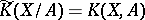. This element is independent of the choice of the trivialization ofabove all of. In the general case one chooses a bundleoversuch that the bundleis trivial, and the triple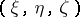is assigned the same element as the triple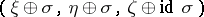.